# Formatting-date-superscript-to-date-value day month year

Wikitechy | 2651 Views | javascript | 04 Jun 2016

• In this format we include a superscript to the date value.
• The idea is to identify the date and then select a superscript based on the date value.
• The sup() method is used to display a string as superscript text.

#### Syntax :

`var sup = "  " ;`

#### Sample Code :

```<html>
<title> Formatting-date </title>
<body>
<script type="text/javascript">
var m_names = new Array("January", "February", "March",
"April", "May", "June", "July", "August", "September",
"October", "November", "December");
var d = new Date();
var curr_date = d.getDate();
var sup = "";
if (curr_date == 1 || curr_date == 21 || curr_date ==31)
{
sup = "st";
}
else if (curr_date == 2 || curr_date == 22)
{
sup = "nd";
}
else if (curr_date == 3 || curr_date == 23)
{
sup = "rd";
}
else
{
sup = "th";
}
var curr_month = d.getMonth();
var curr_year = d.getFullYear();
document.write(curr_date + "<SUP>" + sup + "</SUP> " +
m_names[curr_month] + " " + curr_year);
</script>
</body>
</html>
```

#### Code Explanation :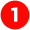Here <script type="text/javascript"> specifies the type attribute of the Internet media type (formerly known as MIME type) of a script. "text/javascript” specifies the media type.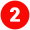Here the var m_names = new Array("January", "February", "March", "April", "May", "June", "July", "August", "September",  "October", "November", "December"); specifies the getMonth() function that gives us the month in a non-numeric form. To convert this value into the month name, we will employ an array. The array would contain all the 12 month names.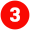var d = new Date () specifies a variable d contains a new date object. We can now call the various methods of this date object.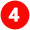var curr_date = d.getDate() it return the current day of the month.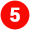var sup = "" specifies to Returns the sup() method is used to display a string as superscript text.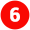We first initialize the variable sup that would store the superscript value. Using if (curr_date == 1 || curr_date == 21 || curr_date ==31), we check the value of the current date and according to that we  assign the  value to sup(super script). (if condition is false it goes to else if condition).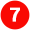sup = "st"; specifies “st” which is used with numbers ending in 1 such as 1st , 21st, 31st pronounced First, Twenty-first, Thirty-first).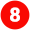We first initialize a variable sup that would store the superscript value. Using else if (curr_date == 2 || curr_date == 22) we check the value of the current date and accordingly we assign the value to sup(super script). (if condition is false it goes to else if condition).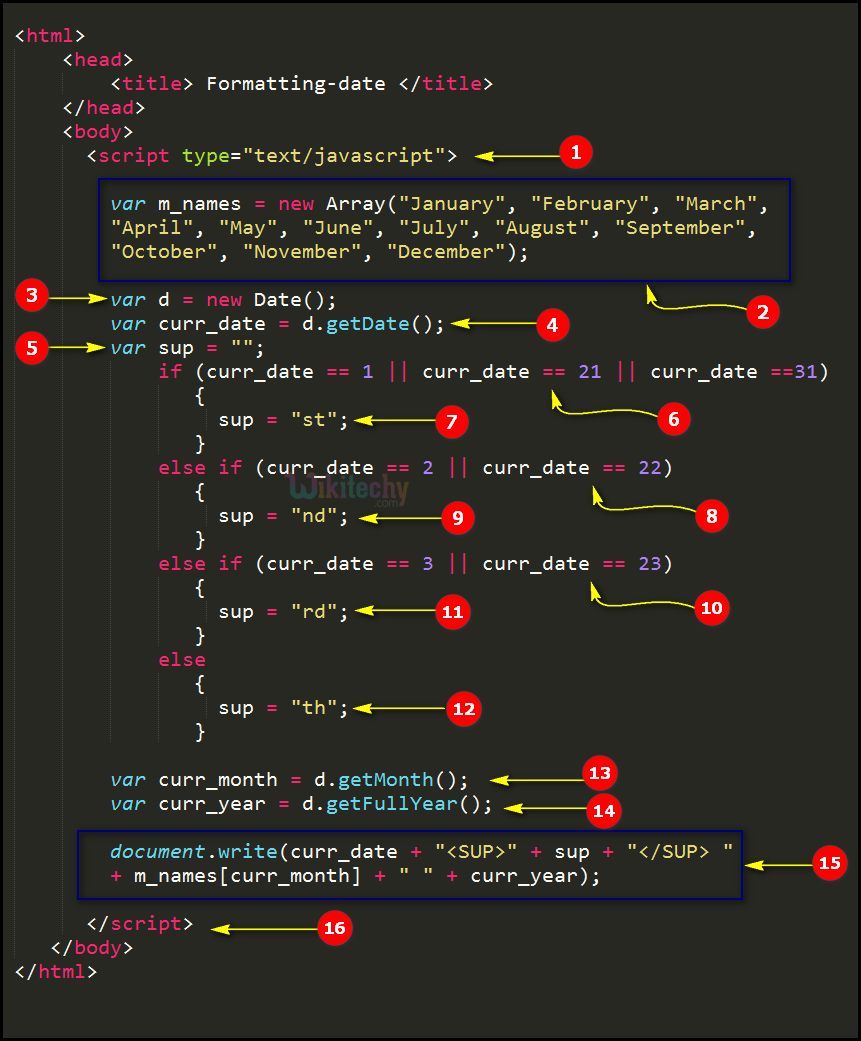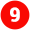sup = "nd"; specifies “nd” which is used with numbers ending in 2 like 2nd, 22nd pronounced second, twenty-second)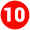We first initialize a variable sup that would store the superscript value. Using else if (curr_date == 3 || curr_date == 23) we check the value of the current date and accordingly we assign the value to sup(super script). (if condition is false it goes to else condition).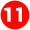sup = "rd"; specifies “rd” which is used with numbers ending in 3 e.g. 3rd ,23rd pronounced third , Twenty-third).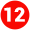sup = "th"; specifies as an exception to the above rules, all the "teen" numbers ending with 11th , 12th .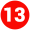var curr_month = d.getMonth() specifies the current month.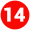var curr_year = d.getFullYear() specifies the method that returns the year (four digits for dates between year 1000 and 9999) of the specified date.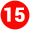document.write specifies the write() method writes JavaScript code to a document.(curr_date+"<SUP>"+sup+"</SUP>"+ m_names [curr_month] + " " + curr_year); it returns the current date with superscript text month name year (like: 30th May 2016) in this format.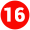</script> defines the end of script tag in the sample code.

## Sample Output :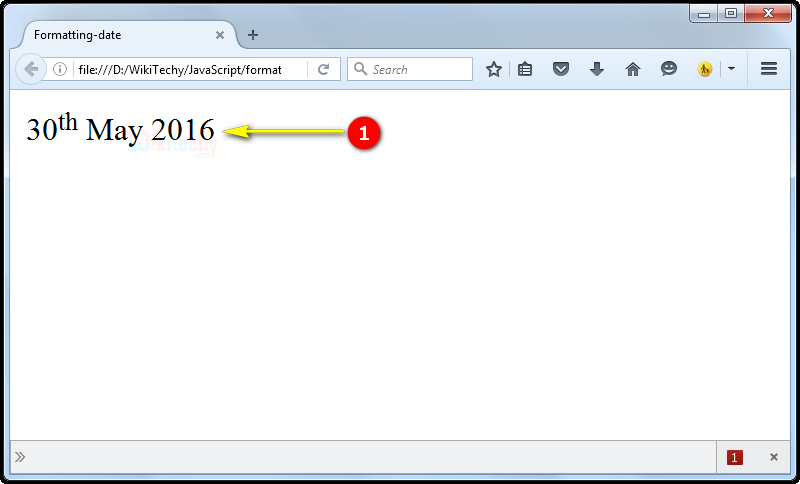Here in this output we display 30th May 2016 which specifies the datesup (date superscript text) month year.

Bug Bounty
Webinar